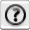# Jeks Display

You must allow Java in your browser to test Jeks Display...

###Introduction

This applet parses a function definition with 1 or 2 parameters of type f(x)=... or g(x,y)=...
After compilation, the results are displayed in a two or three dimensions space, for a number of parameters equal to 1 or 2 respectively (y=f(x) or z=g(x,y)).
This applet is a demo of the features of Jeks library.

###User's guide

Enter a function in the Function text field, with the name of a function followed by one or two parameters between brackets, and by a = sign. After the sign =, enter any expression using the basics operators (+ - * / MOD ^) or classical mathematical functions (LN, LOG, EXP, SQR, SQRT, COS, SIN, TAN, ACOS, ASIN, ATAN, COSH, SINH, TANH, INT, ABS, OPP) ; calculations may operate on numerical values or on the parameters defined with the function.
Example of functions :

• f(a) = a ^ 2
• g(x,y) = cos (x) * sin (y)

Then, validate this function with the <RETURN> key. If there's a syntax error, a message displays the error and the character number where it occurred, otherwise the curve (or the surface) corresponding to the entered function is displayed.
You can modified the Minimum and Maximum limits of the display space. For 3D surfaces, three Mesh modes allows to modify the way the surface is computed :

• Complete mesh : The mesh is displayed with no hidden surfaces computation.
• White mesh : The mesh is displayed in white without hidden surfaces.
• Lighted mesh : The mesh is displayed with lighting and without hidden surfaces.

You can turn the 3D surface, with the mouse : click on the surface and move the mouse pointer while keeping the button pressed. To display the surface in its initial position, click on Default position.
Finally, the Compute text field allows to compute a call to the function with one (or two) given numerical value(s), as showed in this example :

• f(10.3)
• g(0.2E1, -3)

Have fun (or maybe good work) !© Copyrights 1997-20220 eTeks - All rights reserved